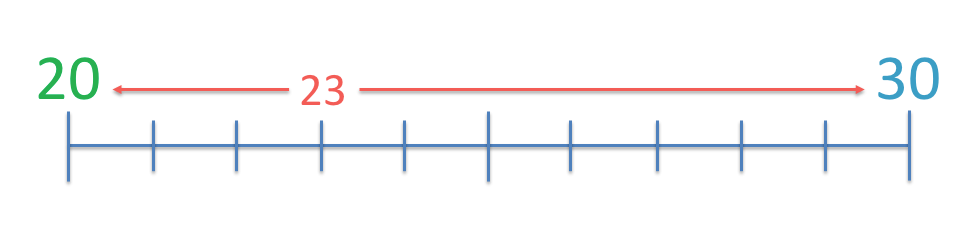# Roman Numerals to 20

Roman Numerals to 20#### Roman Numerals Chart 1 to 10

Before learning the numerals 11 to 20 first watch our lesson on Roman Numerals 1 to 10.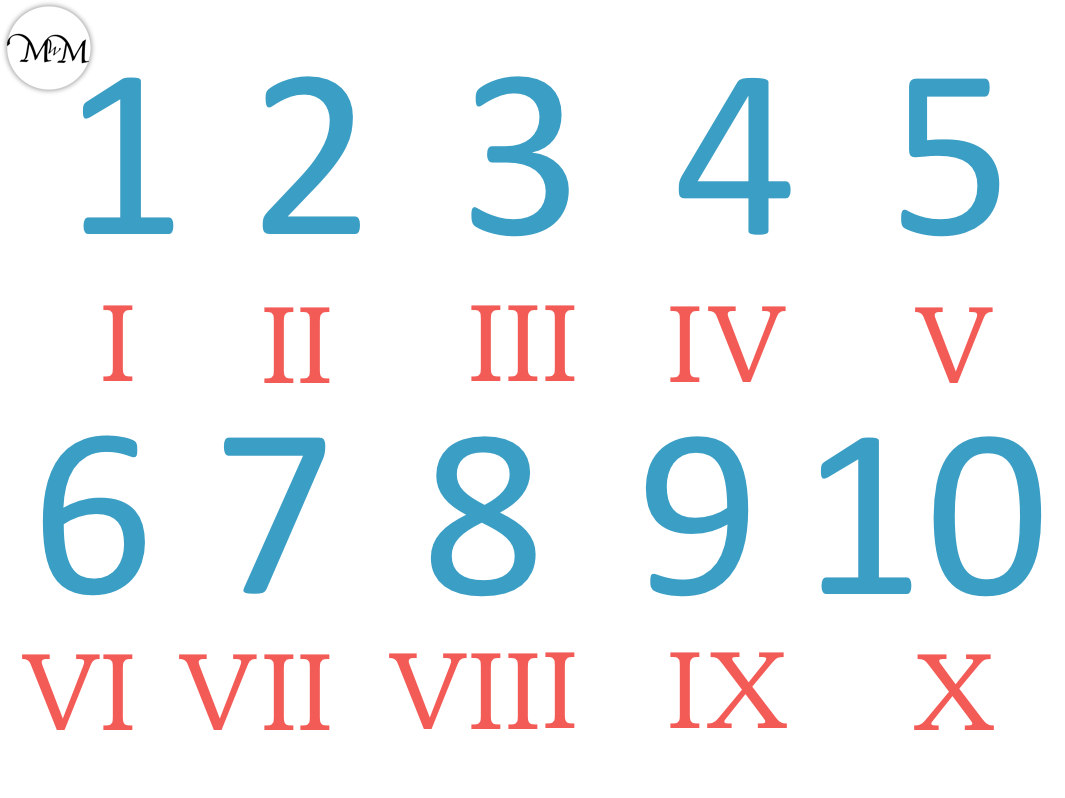#### Roman Numerals Chart 11 to 20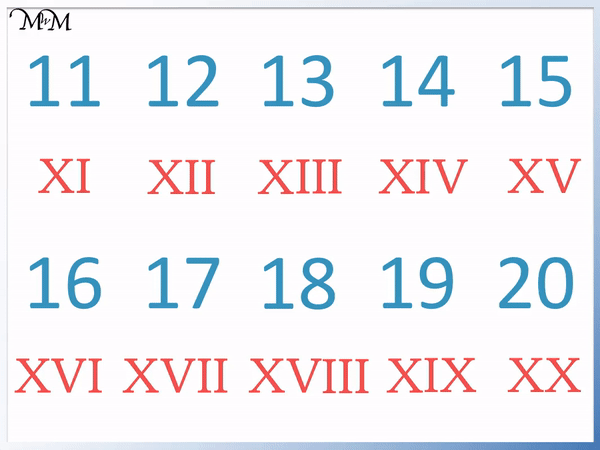• The Roman numerals 11 to 20 are simply ten larger than the Roman numerals 1 to 10.
• We add the numerals for 1-10 after this X.

The Roman numerals 11 to 20 are simply the same as the Roman Numerals 1 to 10 but with an X numeral in front.• XVII is made of the numerals X, V and two I‘s.
• X is worth 10, V is worth 5 and I is worth 1.
• XVII is the same as 10 + 5 + 1 + 1.
• XVII is worth seventeen.
• VII is 5 + 1 + 1, which equals 7.
• If we know that VII is 7, then we can make 17 by simply writing an X numeral in front.#### Roman Numerals to 20 Interactive Question Generator

Roman Numerals to 20: Interactive Questions

# Writing Roman Numerals to 20

The Roman numerals to 20 are made up of the following three numerals.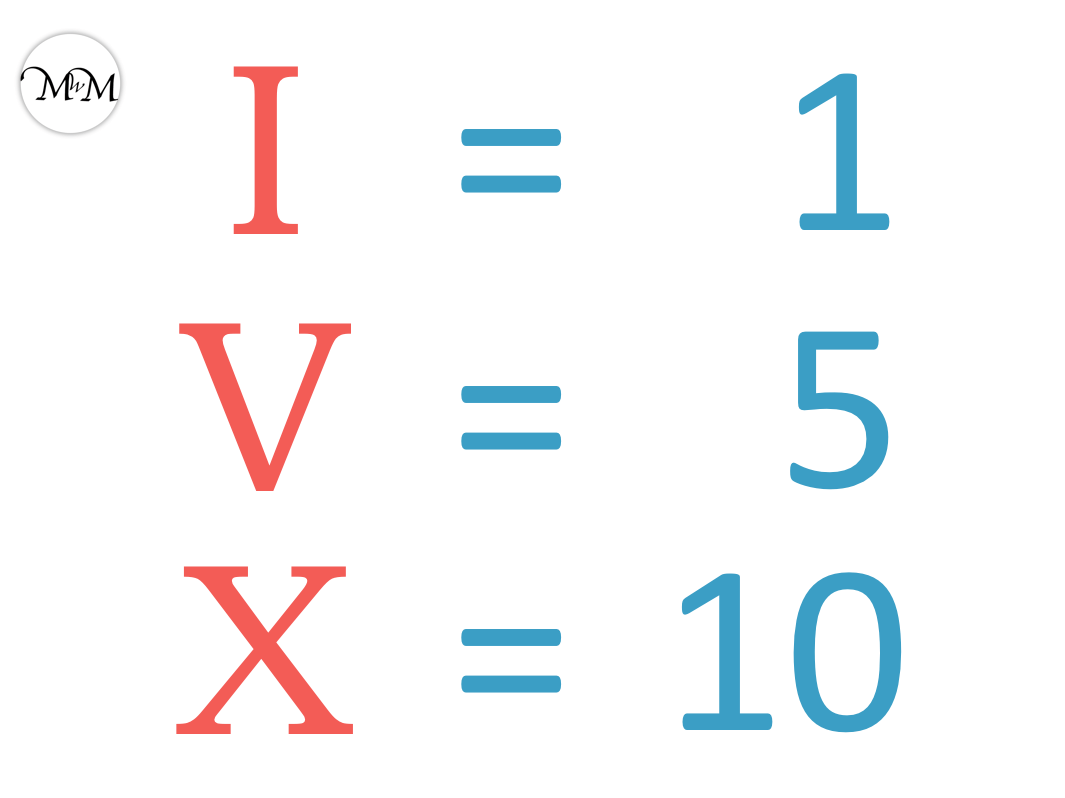• I = 1
• V = 5
• X = 10
• We can add up to three of the same numeral together in a row to make larger numbers.

For example, two I numerals is two lots of one and makes a total of 2.

2 is written as II in Roman numerals.We can write III as 3. This is because we have three I numerals. 1 + 1 + 1 = 3.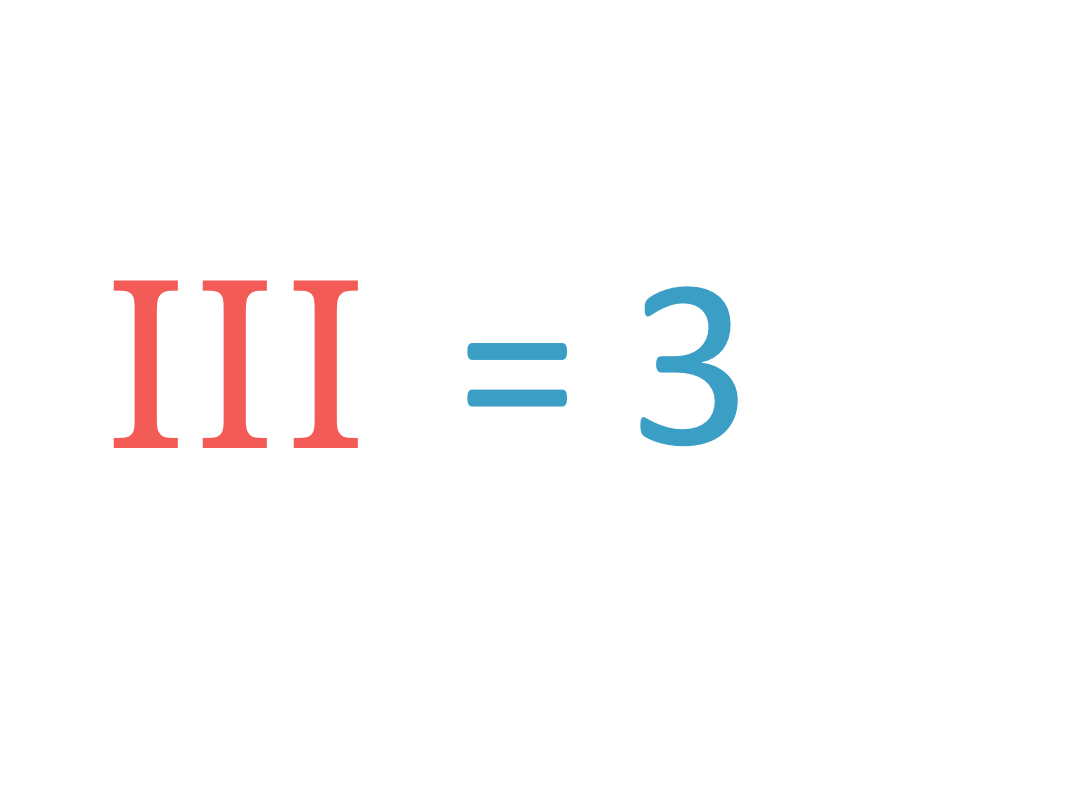We cannot write 4 as IIII because it uses four I numerals. The most we are allowed to use together is three.

Instead we write 4 as IV, which is an I before a V, which means 1 before 5.We can count on from 5 to make larger numbers.

VI means V plus I, which means 5 plus 1.

VI is 6 in Roman numerals.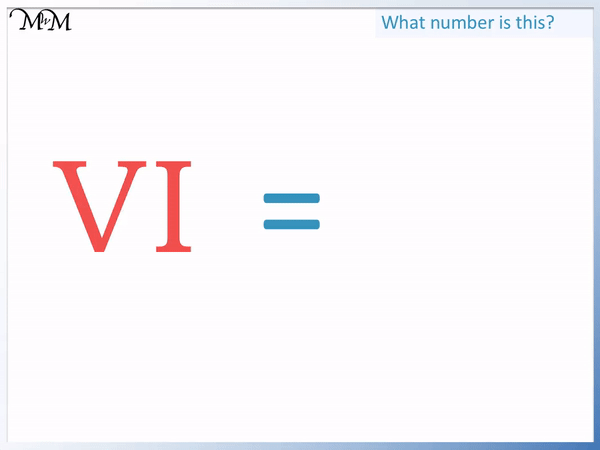Similarly 7 is written as VII because V + I + I means 5 + 1 + 1.

8 is VIII because V + I + I + I means 5 + 1 + 1 + 1.

Again we can’t write 9 as VIIII because it uses four of the same numeral at once. We are only allowed to write three of the same numeral at once.

Instead, 9 is written as 1 before 10. We write IX because I is 1 and X is 10.

Here are the Roman numerals to 10. Please visit our lesson on Roman numerals 1 to 10 for our video lesson. When teaching the Roman numerals to 20, we recommend first learning these numerals to 10.11 is ten more than 1, 12 is ten more than 2, 13 is ten more than 3 and so on.

The numbers 11 to 20 are simply 10 more than the numbers 1 to 10.

To write the Roman numerals to 20, simply add the numerals for the numbers 1 to 10 to the numeral X.

X is worth ten and when writing any numerals after this, it will add ten to those numerals.

Here are the Roman numerals 11 to 20 formed by writing an X numeral in front of the numeral that is 10 less in value.For example, 11 is ten more than 1.

The numeral for 1 is I.

Therefore to write 11, we write an X and then the numeral for 1.

11 is written as XI in Roman numerals.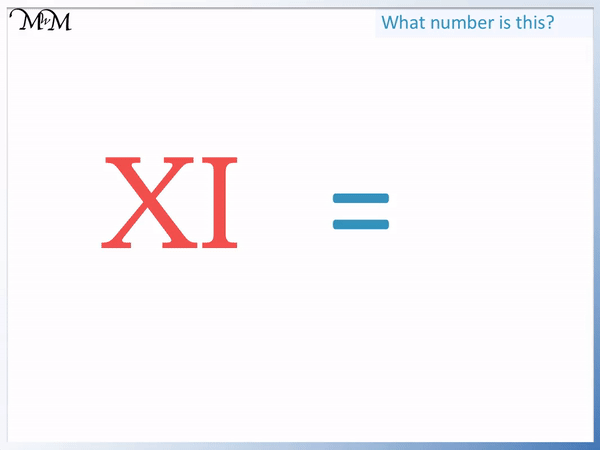XI means X + I, which is 10 + 1.

10 + 1 = 11.

Similarly, 15 is ten more than 5.

Therefore to write 15, we write an X and then the numeral for 5.

The numeral for 5 is V.

15 is written as XV in Roman numerals.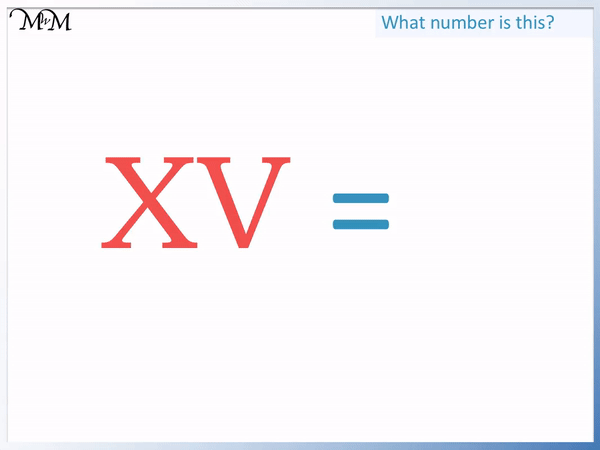XV is X + V, which means 10 + 5.

It is important to note that we always need to write the larger numerals first when adding Roman numerals.

We have to write XV in that order because X is worth 10 and V is only worth 5.

We cannot write V then X as it breaks this rule. We simply write an X and then the smaller value numerals.

Here we have XVII.

This means X + V + I + I, which means 10 + 5 + 1 + 1.

XVII are the Roman numerals for 17.VII is 7 and so, if we remember how to write 7, then we simply write an X in front to make 17.

Remember that 4 is written as IV because we can’t use four I numerals.

IV is one before five, which is four.

XIV is simply IV with an X numeral before it.

XIV is X + IV, which means 10 + 4.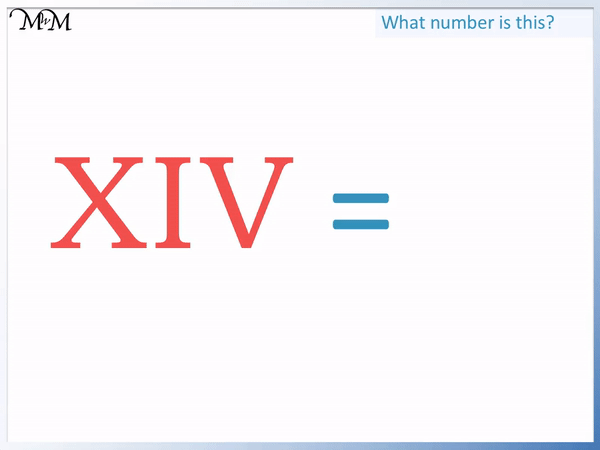Remember that we only add Roman numerals when they are decreasing in size.

I is less than V and so we do not add IV to make 6.

Instead we subtract I from V to make 4. It is easiest to read IV as 1 before 5.

6 is 1 after five, which is written as VI.

Here is the final list of the Roman numerals for 11 to 20. We can see the numeral X highlighted in blue, with the numerals following shown in red.Now try our lesson on Rounding Off to the Nearest 10 on a Number Line where we learn how to round numbers to the nearest ten.